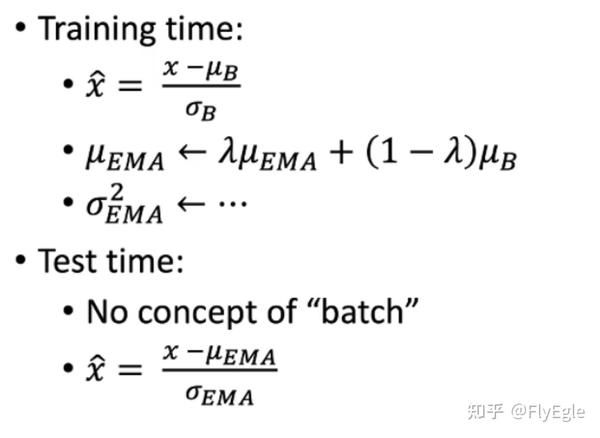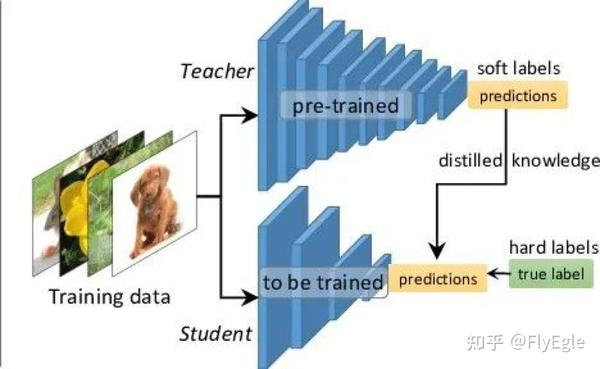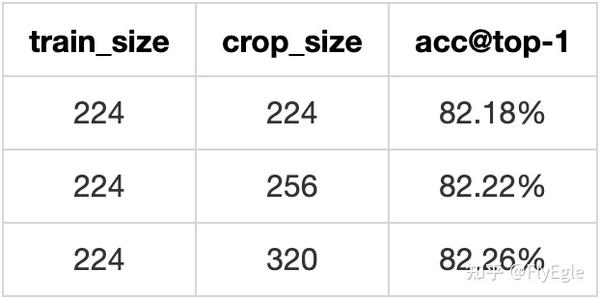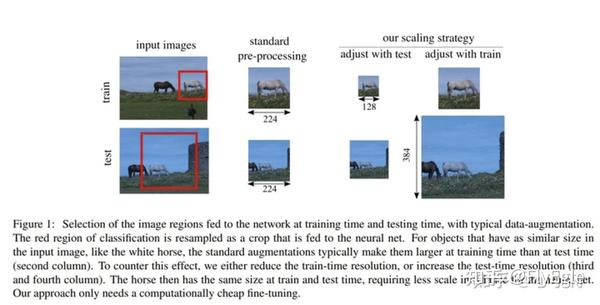• 问答
• 技术
• 实践
• 资源

## 二、Tricks

• 权重平均
• 蒸馏
• 分辨率

### 1. EMA

EMA: $v{t} = \beta \cdot v{t-1} + (1 - \beta) \cdot x{t}$,其中，$v{t}$表示前 $t$条的平均值 ( $v_{0}=0$)， $\beta$是加权权重值 (一般设为0.9-0.999)。

class ModelEma(nn.Module):
def __init__(self, model, decay=0.9999, device=None):
super(ModelEma, self).__init__()
# make a copy of the model for accumulating moving average of weights
self.module = deepcopy(model)
self.module.eval()
self.decay = decay
self.device = device  # perform ema on different device from model if set
if self.device is not None:
self.module.to(device=device)

def _update(self, model, update_fn):
for ema_v, model_v in zip(self.module.state_dict().values(), model.state_dict().values()):
if self.device is not None:
model_v = model_v.to(device=self.device)
ema_v.copy_(update_fn(ema_v, model_v))

def update(self, model):
self._update(model, update_fn=lambda e, m: self.decay * e + (1. - self.decay) * m)

def set(self, model):
self._update(model, update_fn=lambda e, m: m)

EMA的好处是在于不需要增加额外的训练时间，也不需要手动调参，只需要在测试阶段，多进行几组测试挑选最好偶的结果即可。不过是否真的具有提升，还是和具体任务相关，比赛的话可以多加尝试。

### 2. SWA

• SWA可以改进模型训练过程的稳定性；
• SWA的扩展方法可以达到高精度的贝叶斯模型平均的效果，同时对深度学习模型进行校准；
• 即便是在低精度(int8)下训练的SWA，即SWALP，也可以达到全精度下SGD训练的效果。

from torchcontrib.optim import SWA

...
...

# training loop
base_opt = torch.optim.SGD(model.parameters(), lr=0.1)
opt = torchcontrib.optim.SWA(base_opt, swa_start=10, swa_freq=5, swa_lr=0.05)
for _ in range(100):
loss_fn(model(input), target).backward()
opt.step()
opt.swap_swa_sgd()

SWA能够work的关键有两点：

1. SWA采用改良的学习率策略以便SGD能够继续探索能使模型表现更好的参数空间。比如，我们可以在训练过程的前75\%阶段使用标准的学习率下降策略，在剩下的阶段保持学习率不变。
2. 将SGD经过的参数进行平均。比如，可以将每个epoch最后25\%训练时间的权重进行平均。

class AveragedModel(Module):
def __init__(self, model, device=None, avg_fn=None):
super(AveragedModel, self).__init__()
self.module = deepcopy(model)
if device is not None:
self.module = self.module.to(device)
self.register_buffer('n_averaged',
torch.tensor(0, dtype=torch.long, device=device))
if avg_fn is None:
def avg_fn(averaged_model_parameter, model_parameter, num_averaged):
return averaged_model_parameter + \
(model_parameter - averaged_model_parameter) / (num_averaged + 1)
self.avg_fn = avg_fn

def forward(self, *args, **kwargs):
return self.module(*args, **kwargs)

def update_parameters(self, model):
# p_model have not been done
for p_swa, p_model in zip(self.parameters(), model.parameters()):
device = p_swa.device
p_model_ = p_model.detach().to(device)
if self.n_averaged == 0:
p_swa.detach().copy_(p_model_)
else:
p_swa.detach().copy_(self.avg_fn(p_swa.detach(), p_model_,
self.n_averaged.to(device)))
self.n_averaged += 1

$a{t} = a{t-1} + \frac{x{t} - a{t-1}}{n+1} \$$a{t} = \frac{1}{n+1} \cdot ({x{t-1} + a_{t-1}\cdot n}) \ 由于SWA平均的权重在训练过程中是不会用来预测的，所以当使用opt.swap_swa_sgd()重置权重之后，BN层相对应的统计信息仍然是之前权重的， 所以需要进行一次更新，代码如下： opt.bn_update(train_loader, model) 这里可以引出一个关于bn的小trick ### 3. precise bn 由于BN在训练和测试的时候，mean和std的更新是不一致的，如下图：可以认为训练的时候和我们做aug是类似的，增加“噪声”， 使得模型可以学到的分布变的更广。但是EMA并不是真的平均，如果数据的分布差异很大，那么就需要重新计算bn。简单的做法如下： • 训练一个epoch后，固定参数 • 然后将训练数据输入网络做前向计算，保存每个step的均值和方差。 • 计算所有样本的均值和方差。 • 测试。 代码如下： def update_bn_stats(args: Any, model: nn.Module, data_loader: Iterable[Any], num_iters: int = 200 # pyre-ignore ) -> None: bn_layers = get_bn_modules(model) if len(bn_layers) == 0: return momentum_actual = [bn.momentum for bn in bn_layers] if args.rank == 0: a = [round(i.running_mean.cpu().numpy().max(), 4) for i in bn_layers] logger.info('bn mean max, %s', max(a)) logger.info(a) a = [round(i.running_var.cpu().numpy().max(), 4) for i in bn_layers] logger.info('bn var max, %s', max(a)) logger.info(a) for bn in bn_layers: bn.momentum = 1.0 running_mean = [torch.zeros_like(bn.running_mean) for bn in bn_layers] running_var = [torch.zeros_like(bn.running_var) for bn in bn_layers] ind = -1 for ind, inputs in enumerate(itertools.islice(data_loader, num_iters)): with torch.no_grad(): model(inputs) for i, bn in enumerate(bn_layers): # Accumulates the bn stats. running_mean[i] += (bn.running_mean - running_mean[i]) / (ind + 1) running_var[i] += (bn.running_var - running_var[i]) / (ind + 1) if torch.sum(torch.isnan(bn.running_mean)) > 0 or torch.sum(torch.isnan(bn.running_var)) > 0: raise RuntimeError( "update_bn_stats ERROR(args.rank {}): Got NaN val".format(args.rank)) if torch.sum(torch.isinf(bn.running_mean)) > 0 or torch.sum(torch.isinf(bn.running_var)) > 0: raise RuntimeError( "update_bn_stats ERROR(args.rank {}): Got INf val".format(args.rank)) if torch.sum(~torch.isfinite(bn.running_mean)) > 0 or torch.sum(~torch.isfinite(bn.running_var)) > 0: raise RuntimeError( "update_bn_stats ERROR(args.rank {}): Got INf val".format(args.rank)) assert ind == num_iters - 1, ( "update_bn_stats is meant to run for {} iterations, " "but the dataloader stops at {} iterations.".format(num_iters, ind) ) for i, bn in enumerate(bn_layers): if args.distributed: all_reduce(running_mean[i], op=ReduceOp.SUM) all_reduce(running_var[i], op=ReduceOp.SUM) running_mean[i] = running_mean[i] / args.gpu_nums running_var[i] = running_var[i] / args.gpu_nums bn.running_mean = running_mean[i] bn.running_var = running_var[i] bn.momentum = momentum_actual[i] if args.rank == 0: a = [round(i.cpu().numpy().max(), 4) for i in running_mean] logger.info('bn mean max, %s (%s)', max(a), a) a = [round(i.cpu().numpy().max(), 4) for i in running_var] logger.info('bn var max, %s (%s)', max(a), a) ### 2.2 蒸馏模型蒸馏是一个老生常谈的话题了，不过经过实验以来，蒸馏的确是一个稳定提升性能的技巧，不过这里的性能一般是指小模型来说。如果你的任务是不考虑开销的，直接怼大模型就好了，蒸馏也不需要。但是反之，如果线上资源吃紧，要求FLOPs或者Params，那么蒸馏就是一个非常好的选择。 举个例子，以前每次学渣考试都是60分，学霸考试都是90分，这一次学渣通过抄袭学霸，考到了75分，学霸依然是90分，至于为什么学渣没有考到90分，可能是因为学霸改了答案也可能是因为学霸的字写的好。那么这个抄袭就是蒸馏，但是学霸的知识更丰富，所以分数依然很高，那这个就是所谓的模型泛华能力也叫做鲁棒性 简而言之，蒸馏就是使得弱者逼近强者的手段。这里的弱者被叫做Student模型，强者叫做Teacher模型。 使用蒸馏最好是同源数据或者同源模型，同源数据会防止由于数据归纳的问题发生偏置，同源模型抽取信息特征近似，可以更好的用于KL散度的逼近。 蒸馏过程 • 先训练一个teacher模型，可以是非常非常大的模型，只要显存放的下就行，使用常规CrossEntropy损失进行训练。 • 再训练一个student模型，使用CrossEntropy进行训练，同时，把训练好的teacher模型固定参数后得到logits，用来与student模型的logits进行KL散度学习。 KL散度是一种衡量两个分布之间的匹配程度的方法。定义如下：$$ D{KL}(p||q) = \sum{i=1}^{N}p(x{i})log(\frac{p(x{i})}{q(x_{i})})) \$$其中，$q(x)$是近似分布，$p(x)$是我们想要用$q(x)\$匹配的真实分布。如果两个分布是完全相同的，那么KL为0，KL 散度越小，真实分布与近似分布之间的匹配就越好。

KL散度代码如下：

class KLSoftLoss(nn.Module):
r"""Apply softtarget for kl loss

Arguments:
reduction (str): "batchmean" for the mean loss with the p(x)*(log(p(x)) - log(q(x)))
"""
def __init__(self, temperature=1, reduction="batchmean"):
super(KLSoftLoss, self).__init__()
self.reduction = reduction
self.eps = 1e-7
self.temperature = temperature
self.klloss = nn.KLDivLoss(reduction=self.reduction)

def forward(self, s_logits, t_logits):
s_prob = F.log_softmax(s_logits / self.temperature, 1)
t_prob = F.softmax(t_logits / self.temperature, 1)
loss = self.klloss(s_prob, t_prob) * self.temperature * self.temperature
return loss


### 2.3 分辨率"""
R50 为例子，这里冻结除了最后一个block的bn以及fc以外的所有参数
"""
if args.fixres:
# forzen others layers except the fc
for name, child in model.named_children():
if 'fc' not in name:
for _, params in child.named_parameters():

if args.fixres:
model.eval()
model.module.layer4.bn3.train()

# data aug for fixres train
if self.fix_crop:
self.data_aug = imagenet_transforms.Compose(
[
Resize(int((256 / 224) * self.crop_size)),
imagenet_transforms.CenterCrop(self.crop_size),
imagenet_transforms.ToTensor(),
imagenet_transforms.Normalize(mean=self.mean, std=self.std)
]
)

• 先固定除了最后一层的bn以及FC以外的所有参数。
• 训练的数据增强采用推理的增强方法，crop尺寸和推理大小保持一致。
• 用1e-3的学习率开始进行finetune。

## 三、总结

• EMA, SWA基本上都不会影响训练的速度，还可能提点，建议打比赛大家都用起来，毕竟提升0.01都很关键。做业务的话可以不用太care这个东西。
• precise bn， 如果数据的分布差异很大的话，最好还是使用一下，不过会影响训练速度，可以考虑放到最后几个epoch再使用。
• 蒸馏，小模型都建议使用，注意一下调参即可，也只有一个参数，多试试就行了。
• FixRes，固定FLOPs的场景或者想突破精度都可以使用，简单有效。

•0
•0
•174收藏

• 41

关注
• 123

获赞
• 9

精选文章

• 人脸识别方向

• 人脸识别

• 69
• 73
• 81
• 72
• 170